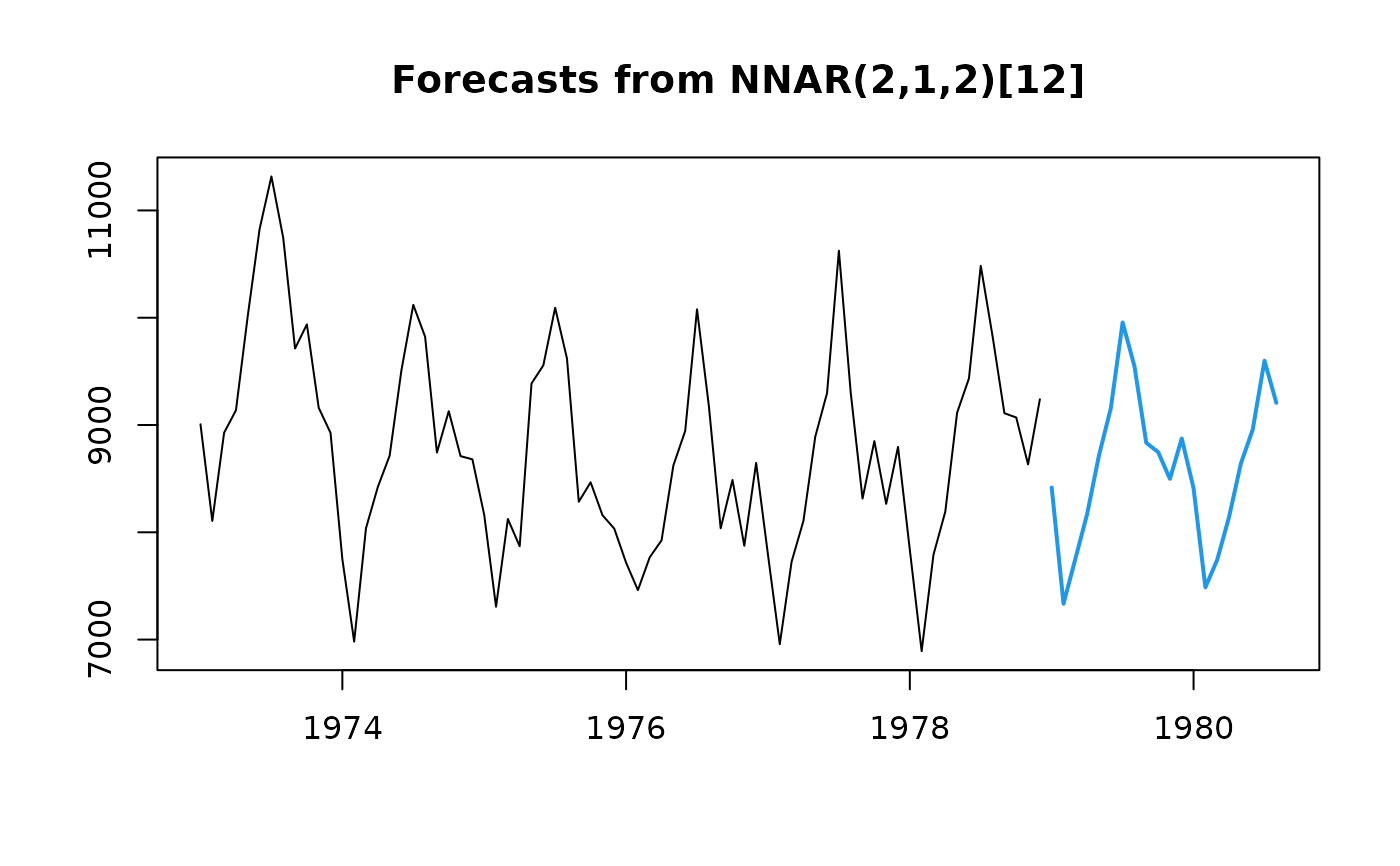Returns forecasts and other information for univariate neural network models.

# S3 method for nnetar
forecast(
object,
h = ifelse(object$m > 1, 2 * object$m, 10),
PI = FALSE,
level = c(80, 95),
fan = FALSE,
xreg = NULL,
lambda = object$lambda, bootstrap = FALSE, npaths = 1000, innov = NULL, ... ) ## Arguments object An object of class "nnetar" resulting from a call to nnetar. Number of periods for forecasting. If xreg is used, h is ignored and the number of forecast periods is set to the number of rows of xreg. If TRUE, prediction intervals are produced, otherwise only point forecasts are calculated. If PI is FALSE, then level, fan, bootstrap and npaths are all ignored. Confidence level for prediction intervals. If TRUE, level is set to seq(51,99,by=3). This is suitable for fan plots. Future values of external regressor variables. Box-Cox transformation parameter. If lambda="auto", then a transformation is automatically selected using BoxCox.lambda. The transformation is ignored if NULL. Otherwise, data transformed before model is estimated. If TRUE, then prediction intervals computed using simulations with resampled residuals rather than normally distributed errors. Ignored if innov is not NULL. Number of sample paths used in computing simulated prediction intervals. Values to use as innovations for prediction intervals. Must be a matrix with h rows and npaths columns (vectors are coerced into a matrix). If present, bootstrap is ignored. Additional arguments passed to simulate.nnetar ## Value An object of class "forecast". The function summary is used to obtain and print a summary of the results, while the function plot produces a plot of the forecasts and prediction intervals. The generic accessor functions fitted.values and residuals extract useful features of the value returned by forecast.nnetar. An object of class "forecast" is a list containing at least the following elements: model A list containing information about the fitted model method The name of the forecasting method as a character string mean Point forecasts as a time series lower Lower limits for prediction intervals upper Upper limits for prediction intervals level The confidence values associated with the prediction intervals x The original time series (either object itself or the time series used to create the model stored as object). xreg The external regressors used in fitting (if given). residuals Residuals from the fitted model. That is x minus fitted values. fitted Fitted values (one-step forecasts) ... Other arguments ## Details Prediction intervals are calculated through simulations and can be slow. Note that if the network is too complex and overfits the data, the residuals can be arbitrarily small; if used for prediction interval calculations, they could lead to misleadingly small values. It is possible to use out-of-sample residuals to ameliorate this, see examples. ## See also nnetar. ## Author Rob J Hyndman and Gabriel Caceres ## Examples ## Fit & forecast model fit <- nnetar(USAccDeaths, size=2) fcast <- forecast(fit, h=20) plot(fcast)if (FALSE) { ## Include prediction intervals in forecast fcast2 <- forecast(fit, h=20, PI=TRUE, npaths=100) plot(fcast2) ## Set up out-of-sample innovations using cross-validation fit_cv <- CVar(USAccDeaths, size=2) res_sd <- sd(fit_cv$residuals, na.rm=TRUE)
myinnovs <- rnorm(20*100, mean=0, sd=res_sd)
## Forecast using new innovations
fcast3 <- forecast(fit, h=20, PI=TRUE, npaths=100, innov=myinnovs)
plot(fcast3)
}